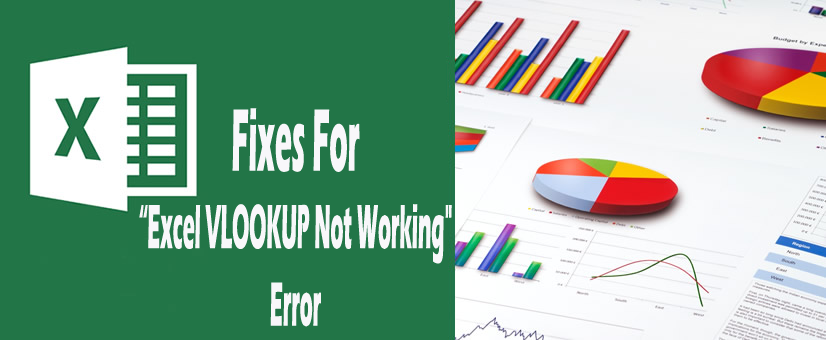Troubleshooting problems regarding Excel worksheets formula and functions can be a big job. Actually, the error comes with the package. Though Excel offers some auditing tools, which is found in formulas tab in the Formula Auditing group, you don’t always need so much power to fix these relative issues. Sometimes just a bit of Excel special knowledge can help you to resolve issue occurred with such Excel formulas and functions. This tutorial explains how you can quickly cope with Excel 2013, 2010, 2007 and 2003, “VLOOKUP not working issue. So, that you can easily troubleshoot and fix common errors and overcome VLOOKUP’s limitations also.

Users of the VLOOKUP, HLOOKUP or MATCH functions sometimes get an unexpected #N/A  error when they try to match a lookup value within an array. This error generally indicates that the function has actually failed to find the lookup value within the lookup array. But what if you can see that the matching value is present there in the lookup array but Excel still doesn’t find this value?

If your function fails to find this match (signaled by the #N/A error), this may be because Excel does not consider the two values to be exactly equal. You can test for this problem via the following steps:

The VLOOKUP function is popular lookup and reference function of Excel. It will show tricky and dreaded #N/A error message.

The article will look at the 5 most common reasons why vlookup not working in Excel.

Have a look…

1. You Need an Exact Match
2. Lock the Table Reference
3. A Column Has Been Inserted
4. The Table has got Bigger
5. VLOOKUP Cannot Look to its Left

Also Read:

### 1. You Need an Exact Match

The ending argument of VLOOKUP function, well known as range_lookup, you can search for the approximate or an exact match.

In most cases, users look for particular product order, employee or customer and therefore requires and an exact match. if you are searching for any unique value, enter FALSE for the range_lookup argument.

This one is optional, but if it is left unfilled then the TRUE value must be used.  The TRUE value relies on your data is sorted in order to work.

The Below shown figure will shows a VLOOKUP with the range_lookup argument omitted and the incorrect value being returned.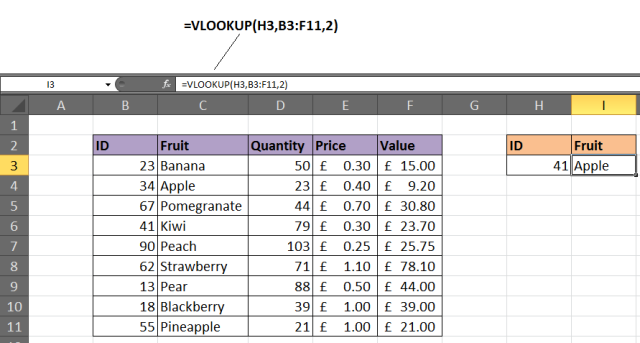#### Solution

If looking for some unique value then enter FALSE value for the ending argument. The VLOOKUP must be entered like =VLOOKUP(H3,B3: F11,2,FALSE).

This is the first reason behind the vlookup not working in Excel.

### 2. Lock the Table Reference

If you are using multiple VLOOKUPs in excel to catch different information about any particular records. or if in case you are willing to copy off your VLOOKUP to several of your cells then you have to check your table.

Below shown image shows that the VLOOKUP entered over here is incorrect. The incorrect cell ranges are referenced for the lookup_value and table array.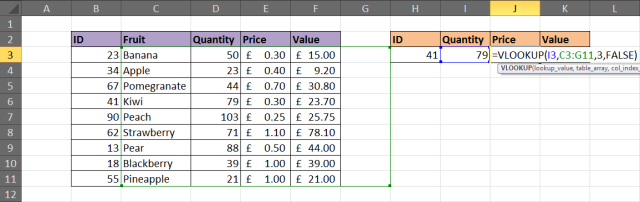#### Solution

The table which is used by VLOOKUP function actually search for and gives information from is called astable_array. This is required to get referenced completely in order to copy your VLOOKUP.

So, click on the references which are right inside the formula and after then press the F4 key on your keyboard to modify the reference from relative to absolute. So,  enter the formula  as=VLOOKUP(\$H\$3,\$B\$3:\$F\$11,4,FALSE).

In this example, both the lookup_value and table_array references made was absolute. Basically it may be the table_array that needs locking.

### 3. To Insert A Column

The column index number or col_index_num that is used by the VLOOKUP function in order to enter information to return a record.

Because this is entered as an index number, and it is not that durable one. If a column is putted down in the table, then vlookup is not working as this stop VLOOKUP from working. Here is the image shown below for a scenario.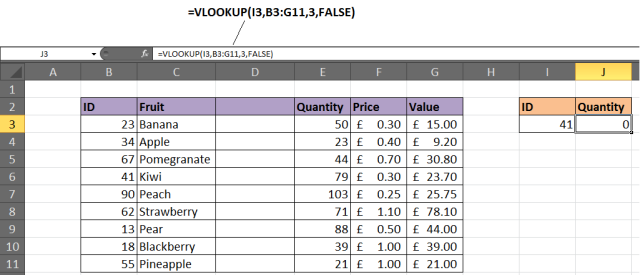The whole content is in column 3, but after the insertion of the new column, it became column 4.  However, the VLOOKUP has not automatically updated.

#### Solution 1

One solution you can try to save your worksheet so any other user can’t insert columns. If the user requires to do so, then it’s not a valid solution.

#### Solution 2

Another option is to insert the MATCH function within the col_index_num argument of VLOOKUP.

The MATCH function can be used to search for something or returning the required column number. This will; makes the col_index_num dynamic so that the inserted columns won’t affect the VLOOKUP.

The formula given below needs to be entered within this example to avoid above mentioned problem.

### 4. The Table has got Bigger

When more rows get added into the table, vlookup requires to get updated. Below given image shows that vlookup don’t check complete table fruit items.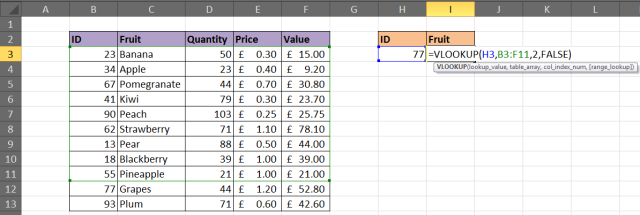#### Solution

You can consider the formatting range as dynamic range name or table (Excel 2007+). This process ensures that vlookup will look over complete of your table.

In order to format the range as table, choose the cells range that you want to use for table_array. After then tap to the Home Format as Table and choose any one style from gallery section. Tap to the Design tab present within Table Tools and then change the table name in the given box.

The VLOOKUP below shows a table named FruitList being used.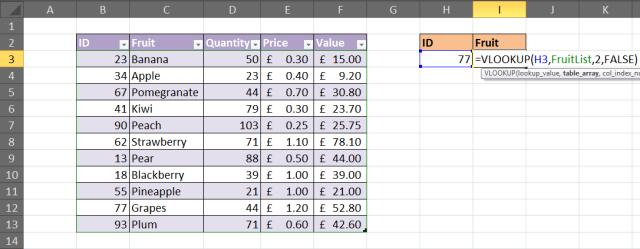### 5. VLOOKUP Can’t Look On It’s Left

Limitation of the VLOOKUP function is that it can’t look at its left. This will appear at the left most column of a table and return information from the right.

#### Solution

Solution to fix the VLOOKUP not working issue is not to use VLOOKUP at all. You can use the combination of  INDEX and MATCH excel function as an alternative for VLOOKUP.

The below given example is to show what information is returned to the left of the column you are looking in.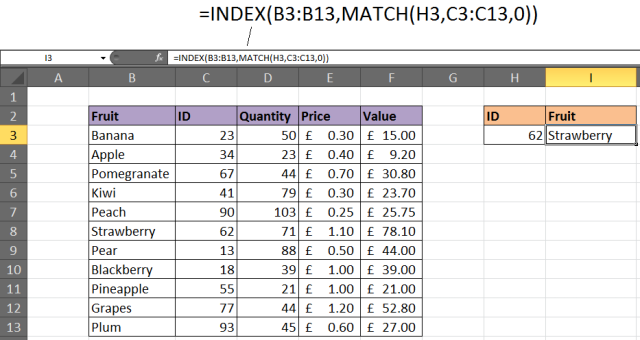These are the 5 Best reason behind VLOOKUP not working error in Excel. Hope now the Excel VLOOKUP not working error is fixed but if not then makes use of the automatic solution to fix the VLOOKUP not working error in Excel

## Automatic Solution: MS Excel Repair Tool

The given manual solution will surely fix off your excel files issues and errors. but if in case you caught into any other corruption glitches or damaged file issue then make use of MS Excel Repair Tool.  it is best suited software for restoring down the corrupt Excel files and also for the retrieval of data from excel worksheet like cell comments, charts, worksheet properties and other stuffs. It’s a professionally designed program that easily repairs off the .xls and .xlsx files.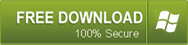### Conclusion

I tried my best to provide the ample information about the VLOOKUP is not working error in Excel. However, if you are having any additional fixes or any query then please share it with us. You can go to the comment section or on MS Excel Ask Question page.

Good Luck…5 Reasons Behind “Excel VLOOKUP Not Working” Error Occurrence
SummaryArticle Name
5 Reasons Behind “Excel VLOOKUP Not Working" Error Occurrence
Description
Are you the one facing Excel VLOOKUP Not Working Error then here know the 5 reasons behind getting the error along with the solution also.
• 11
Shares